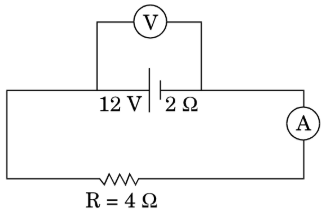NEET Physics Current Electricity Questions Solved

(a) The potential difference applied across a given resistor is altered so

that the heat produced per second increases by a factor of 9. By what

factor does the applied potential difference change?

(b) In figure shown, an ammeter A and a resistor of 4$\mathrm{\Omega }$ are connected

to the terminals of the source. The emf of the source is 12 V having

an internal resistance of 2$\mathrm{\Omega }$. Calculate the voltmeter and ammeter